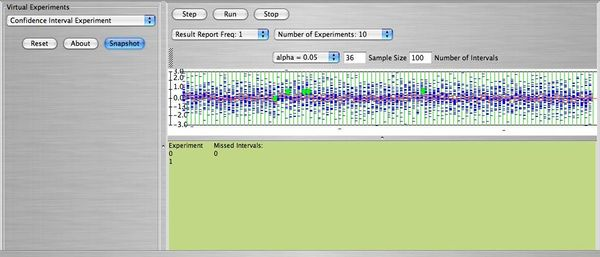# Difference between revisions of "SOCR EduMaterials Activities ConfIntervals"

## This is an activity to explore the confidence intervals for the populatuon mean when the standard deviation is known.

• Description: You can access the applet for the confidence intervals experiment at SOCR Experiments. Use the scroll down button to find the "Confidence Interval Experiment".

The confidence interval for the population mean $$\mu$$ when $$\sigma$$ is known is given by$\bar x - z_{\frac{\alpha}{2}} \frac{\sigma}{\sqrt{n}} \le \mu \le \bar x + z_{\frac{\alpha}{2}} \frac{\sigma}{\sqrt{n}}$ where $$z_{\frac{\alpha}{2}}$$ is the value of $$z$$ such that the area to its left (or right) is $$\frac{\alpha}{2}$$. For example if we choose a $$95 \%$$ confidence level then $$1-\alpha=0.95$$ or $$\alpha=0.05$$ and therefore $$\frac{\alpha}{2}=0.025$$ which gives $$z_{\frac{\alpha}{2}}=1.96$$. The sample mean $$\bar x$$ is the mean of the sample of size $$n$$, and $$\sigma$$ is the standard deviaton. In this lab we will generate many confidence intervals based on different sample sizes. The samples in this lab are always selected from the standard normal distribution $$N(0,1)$$. Therefore we know that the mean is $$\mu=0$$, and the standard deviation $$\sigma=1$$. Let's pretend that $$\mu$$ is unknown and that only $$\sigma$$ is known. We will select many samples each one of size $$n$$ and use it to construct a confidence interval for the population mean.

• Exercise 1:

Using the scroll down button select "Number of Experiments = 100". Select sample size $$n=20$$, and choose number of intervals 200. It means: You will select 200 samples and with each sample you will obtain a confidence interval. You will do this 100 times. How many intervals (out of the 200) do you expect to miss the population mean $$\mu=0$$? Take a snapshot and describe what you observe.

• 1. What do the numbers -3, -2, -1, 0, 1, 2, 3 represent?
• 2. What do the blue lines represent?
• 3. How is the confidence interval represented?
• 4. What does the green dot represent?
• 5. Write down the formula on which the confidence intervals are based and explain why they have different width.
• Exercise 2:
• 1. Reset and repeat (a) with $$\alpha=0.01$$. Take a snapshot and describe what you see.
• 2. Reset and repeat (a) with sample size now $$n=80$$. Take a snapshot and describe in detail what you see.
• 3. Reset and repeat (a) with sample size $$n=80$$ and $$\alpha=1.0E-4$$ (this is $$10^{-4}$$). Take a snapshot and describe in detail what you see.

Below you can see a snapshot of the run of 100 intervals with $$n=36, \ \alpha=0.05$$.